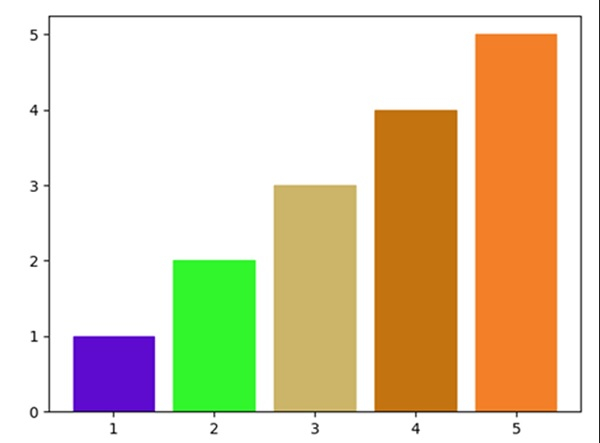# How to give a Pandas/Matplotlib bar graph custom colors?

To make a custom color, we can create a hexadecimal string. From it, we can make different sets of color representation and can pass them into the scatter method to get the desired output.

Using the set_color method, we could set the color of the bar.

## Steps

• Take user input for the number of bars.

• Add bar using plt.bar() method.

• Create colors from hexadecimal alphabets by choosing random characters.

• Set the color for every bar, using set_color() method.

• To show the figure we can use plt.show() method.

## Example

from matplotlib import pyplot as plt
import random

bar_count = int(input("Enter number of bars: "))

bars = plt.bar([i for i in range(1, bar_count+1)], [i for i in range(1, bar_count+1)])

colors = ["#" + ''.join([random.choice(hexadecimal_alphabets) for j in
range(6)]) for i in range(bar_count)]

for i in range(len(colors)):
bars[i].set_color(colors[i])

plt.show()

## Output

Enter number of bars: 5Updated on: 16-Mar-2021

3K+ Views